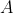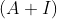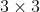# Basis maths-linear algebra-Q19

+1 vote

Letbe a square matrix of order 3 with real entries such that determinant ofis 6 and trace ofis zero. If the determinant of matrixis zero, where I denote theidentity matrix, then one of the eigenvalues ofis _____

(A). 1

(B). 2

(C). -3

(D). 3asked May 15
reshown May 16

+1 voted is correct one because eigen value are -1,-2,3

Solution 2 :hence answer should be option D.answered Jun 11 by (112,390 points)